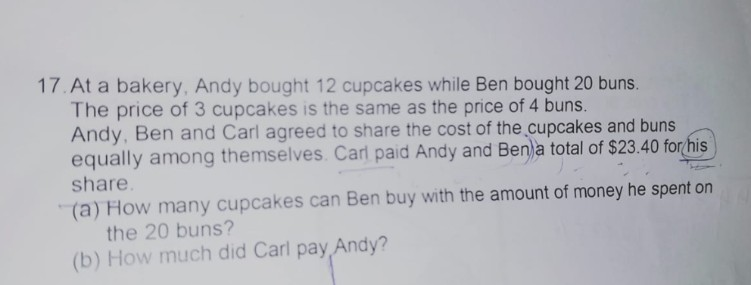# Question1 Answer

# Answer

Suggested answer:

4 buns = 3 cupcakes

20 buns => 3/4 x 20 = 15 cupcakes

a) Ben can buy 15 cupcakes.

C’s share of cost = \$23.40

Total cost = \$23.40 x 3 = \$70.20

3 cupcake = 4 buns

Therefore, 12 cupcake = 16 buns

12 cupcake + 20 buns =16 buns +20 buns = 36 buns

36 buns = \$70.20

1 bun = \$70.20/36 = \$1.95

1 cupcake  = (\$1.95 x 4)/3 = \$2.60

12 cupcakes = \$2.60 x 12 = \$31.20

\$31.20 – \$23.40 = \$7.80

b) Carls paid Andy \$7.80.

0 Replies 1 Like